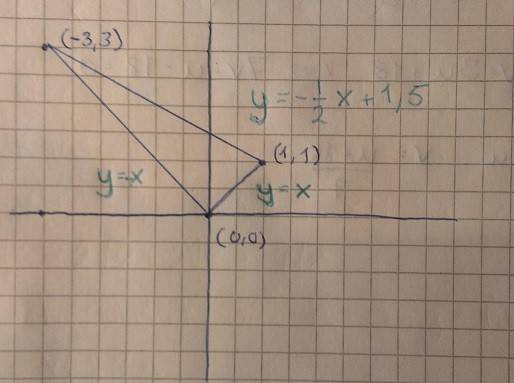# [Double integral] Area of a triangle

Hi! I'm stuck with the following problem:

-----------------------------------
Calculate

∫∫ (x-y)*|ln(x+2y)| dxdy

where D is the triangle with corners in the coordinates (0,0), (1,1) and (-3,3)
-----------------------------------

I get the following lines that forms the triangle: y=-x, y=x and y=-1/2*x+3/2Which gives me ∫∫ u*|ln(v)| dudv

After that I don't know how to continue. I would like to know which new "intervals" I should do the integral between when I am in my new u- and v-domain?